Newton's second law - Examples

Example 1

If you think of acceleration as movement then:

"The greater the mass of the object the more force needed to make it accelerate."

"The greater the mass of the object the more force is needed to make it move."

And this makes sense with the following diagram:Objects with a greater mass have more inertia. It takes more force to change their motion. A 400kg rock is a lot harder to move than a 40kg rock.

Example 2

Force = Push or Pull
Acceleration = Move
Mass = Weight

Be very careful with these ideas because there is a lot more meaning behind each of these words but as a quick rule of thumb it may help jolt a memory.

F (Net force on object) = mass of object xx acceleration

Example 3

Use  F=ma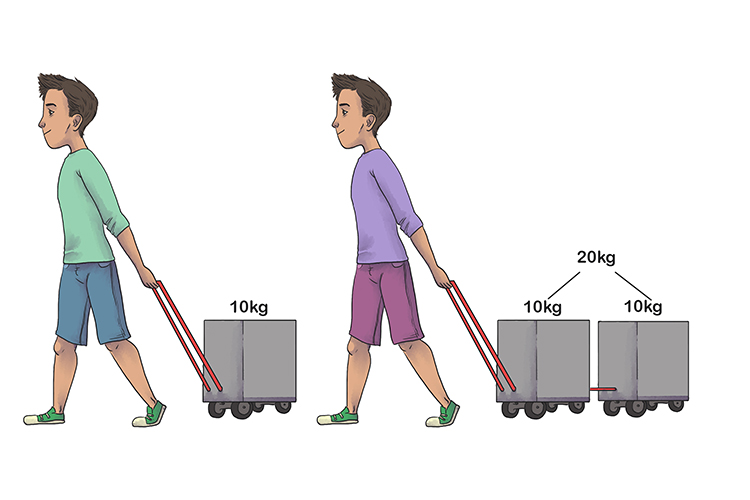It will take twice the amount of force to accelerate the wagon with 20kg as the wagon with 10kg. But in order to make an object accelerate or move you have to apply a force.

Example 4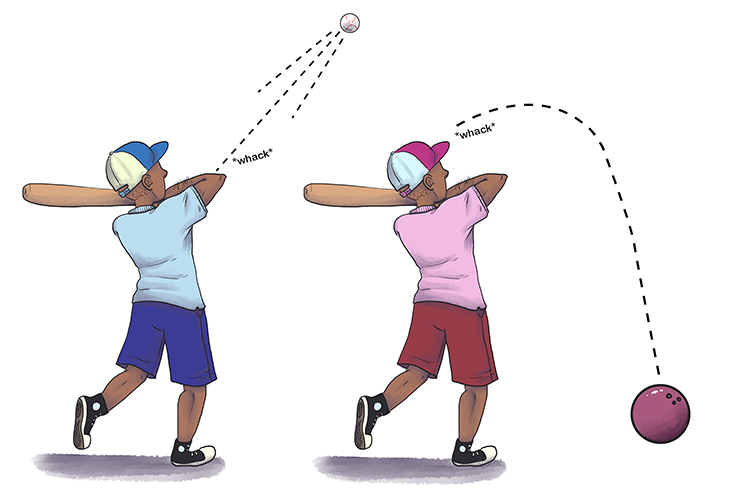Because the mass of each ball is different, each ball will travel a different distance and at a different speed when it is hit with the same force.

Example 5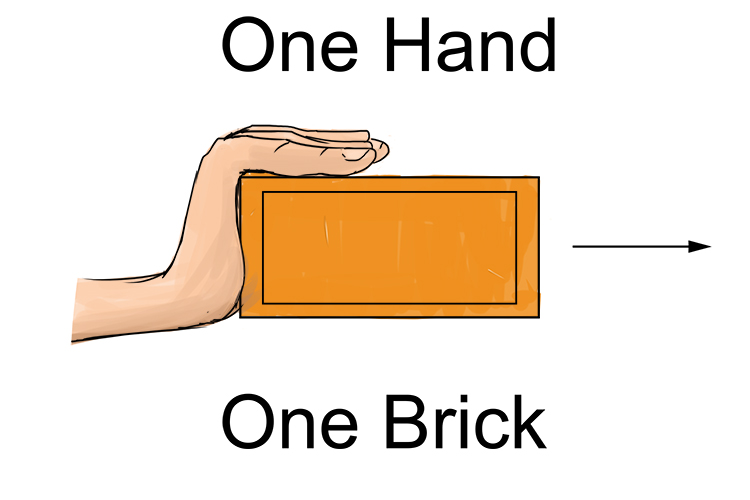Force of hand accelerates the brick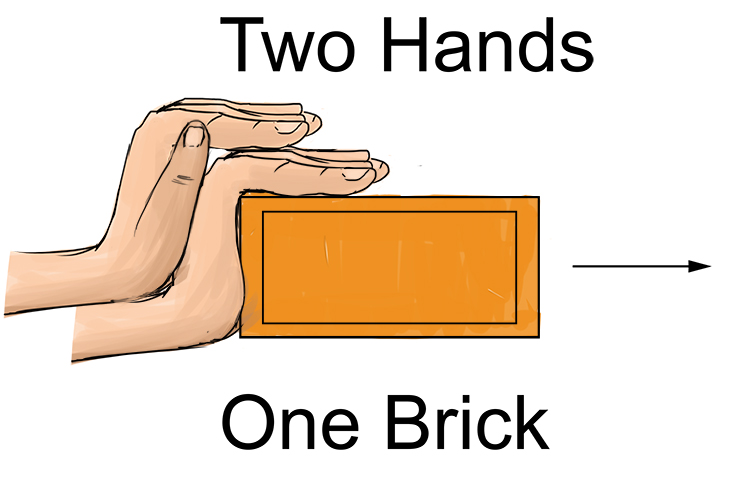Twice as much force produces twice as much acceleration.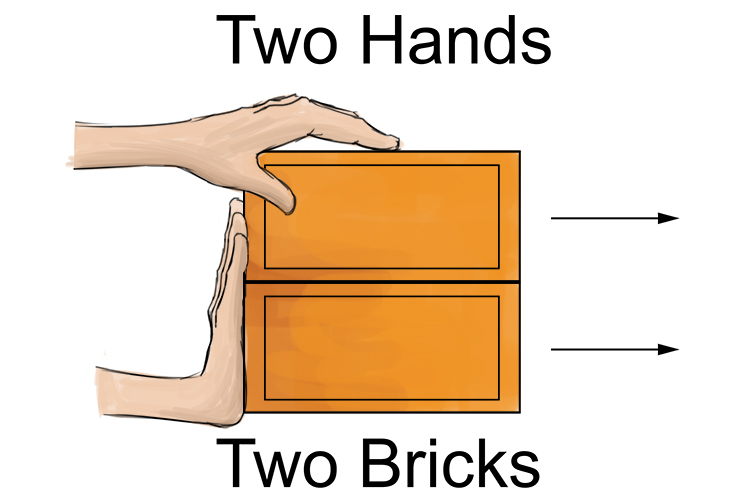Twice the force on twice the mass gives the same acceleration.

Example 6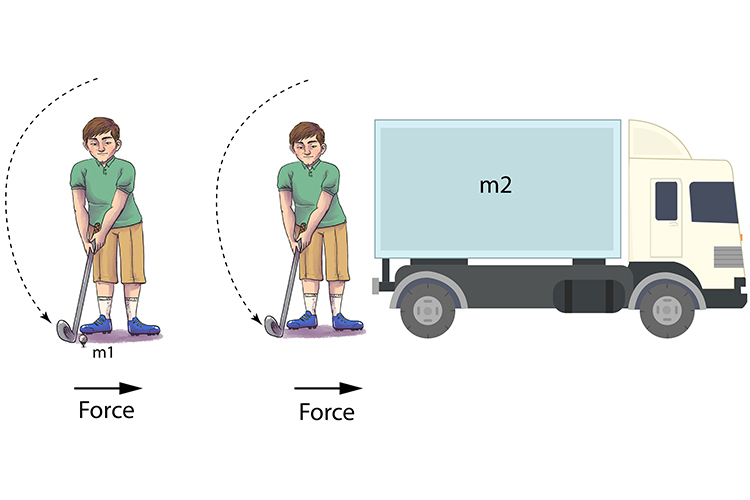F=ma

F=m1xxa Large acceleration (large motion)

F=m2xxa Small acceleration (small motion)

How much an object accelerates depends on the mass of the object and how much force is applied to it.

The effect of a 10 Newton force on a golf ball would be much greater than that same force acting on a truck.

Example 7NET FORCE =0N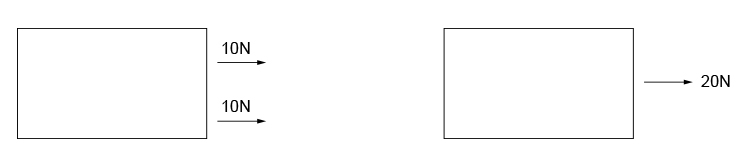NET FORCE =20N

Unbalanced forces cause acceleration.NET FORCE =2N